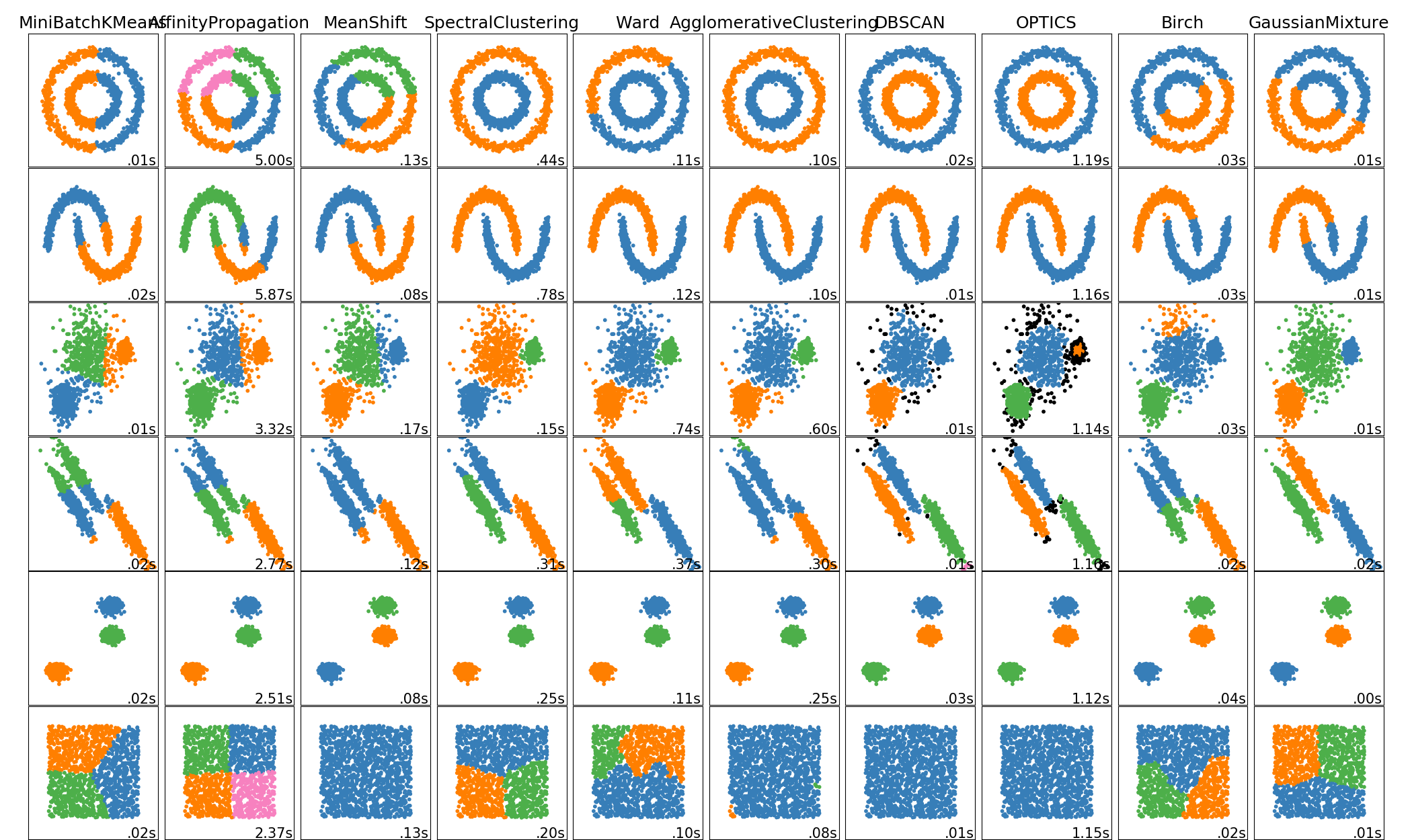# sklearn.cluster.SpectralClustering¶

class sklearn.cluster.SpectralClustering(n_clusters=8, *, eigen_solver=None, n_components=None, random_state=None, n_init=10, gamma=1.0, affinity='rbf', n_neighbors=10, eigen_tol=0.0, assign_labels='kmeans', degree=3, coef0=1, kernel_params=None, n_jobs=None)

[源码]

np.exp(-gamma * d(X,X) ** 2)

n_clusters integer, optional

eigen_solver {None, ‘arpack’, ‘lobpcg’, or ‘amg’}

n_components integer, optional, default=n_clusters

random_state int, RandomState instance, default=None

n_init int, optional, default: 10
k均值算法将使用不同的质心种子运行。最后的结果将是n_init连续运行的最佳inertia输出。
gamma float, default=1.0
rbf，poly, sigmoid, laplacian 和chi2 核的核系数。对于affinity='nearest_neighbors'的情况请忽略。
affinity string or callable, default ‘rbf’

- ‘nearest_neighbors’:通过计算近邻图来构造亲和矩阵。
- ‘rbf’:利用径向基函数(RBF)核构造亲和矩阵。
- ‘precomputed’:将X解释为预先计算的亲和矩阵。
- ‘precomputed_nearest_neighbors’:将X解释为预先计算的近邻的稀疏图，并通过选择n_neighbors近邻构造亲和矩阵。
- 由pairwise_kernels支撑的一种核。

n_neighbors integer

eigen_tol float, optional, default: 0.0
Laplacian矩阵特征点的停止准则， 当eigen_solver='arpack'
assign_labels {‘kmeans’, ‘discretize’}, default: ‘kmeans’

degree float, default=3

coef0 float, default=1

kernel_params dictionary of string to any, optional

n_jobs int or None, optional (default=None)

affinity_matrix_ array-like, shape (n_samples, n_samples)

labels_ array, shape (n_samples,)

np.exp(- dist_matrix ** 2 / (2. * delta ** 2))

Normalized cuts and image segmentation, 2000 Jianbo Shi, Jitendra Malikhttp://citeseer.ist.psu.edu/viewdoc/summary?doi=10.1.1.160.2324

A Tutorial on Spectral Clustering, 2007 Ulrike von Luxburghttp://citeseerx.ist.psu.edu/viewdoc/summary?doi=10.1.1.165.9323

Multiclass spectral clustering, 2003 Stella X. Yu, Jianbo Shihttps://www1.icsi.berkeley.edu/~stellayu/publication/doc/2003kwayICCV.pdf

>>> from sklearn.cluster import SpectralClustering>>> import numpy as np>>> X = np.array([[1, 1], [2, 1], [1, 0],...               [4, 7], [3, 5], [3, 6]])>>> clustering = SpectralClustering(n_clusters=2,...         assign_labels="discretize",...         random_state=0).fit(X)>>> clustering.labels_array([1, 1, 1, 0, 0, 0])>>> clusteringSpectralClustering(assign_labels='discretize', n_clusters=2,    random_state=0)

fit(self, X[, y]) 根据特征或亲和矩阵执行光谱聚类
fit_predict(self, X[, y]) 根据特征或亲和矩阵执行光谱聚类，并返回聚类标签。
get_params(self[, deep]) 获取此估计器的参数
set_params(self, **params) 设置此估计器的参数
__init__(self, n_clusters=8, *, eigen_solver=None, n_components=None, random_state=None, n_init=10, gamma=1.0, affinity='rbf', n_neighbors=10, eigen_tol=0.0, assign_labels='kmeans', degree=3, coef0=1, kernel_params=None, n_jobs=None)

[源码]

fit(self, X, y=None)

[源码]

X array-like or sparse matrix, shape (n_samples, n_features), or array-like, shape (n_samples, n_samples)

y Ignored

self -
fit_predict(self, X, y=None)

[源码]

X array-like or sparse matrix, shape (n_samples, n_features), or array-like, shape (n_samples, n_samples)

y Ignored

labels ndarray, shape (n_samples,)

get_params(self, deep=True)

[源码]

deep bool, default=True

params mapping of string to any

set_params(self, **params)

[源码]

**params dict

self object

## sklearn.cluster.SpectralClustering使用示例¶